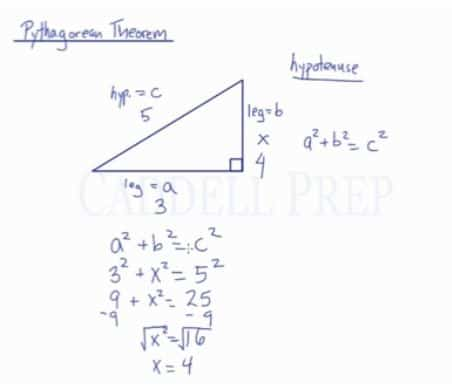In this video, we are going to look at how to use Pythagorean Theorem and how it is used to find a missing length of a side in a right triangle.

Pythagorean Theorem: The square of the hypotenuse is equal to the sum of the squares of the other two sides. This can only be used for right triangles.

The side opposite the right angle is what we call the hypotenuse. The other two sides are what we call the legs. If we label the legs as$a$ and$b$, and the hypotenuse as$c$, then the Pythagorean Theorem states that:$a^2+b^2=c^2$

This can be used to find the missing side of a right triangle.

For example:
If the legs of a triangle are measured in 3 units and 4 units, what is the length of the hypotenuse?

We can substitute these values into the Pythagorean Theorem:$a^2+b^2=c^2$$3^2+4^2=x^2$$9+16=x^2$$25=x^2$$\sqrt{25}=\sqrt{x^2}$$5=x$
The hypotenuse is 5 units long.Video-Lesson Transcript

In this lesson, we’ll review Pythagorean theorem.

It’s very important that the Pythagorean theorem will only be used for right triangles.

Let’s go over this right triangle.

The hypotenuse is the side opposite the right angle.

The other sides which are not hypotenuse, are the legs.

Pythagorean theorem states that$a^2 + b^2 = c^2$

where$a$ is the measure of one of the legs;$b$ is the measure of another leg;
and$c$ is the measure of the hypotenuse

One leg can equal to$a$ or$b$, it doesn’t matter.

But the hypotenuse is always$c$.

It’s always leg squared plus leg squared equal hypotenuse leg.

If we have two measurements, we can always find the third one using this theorem.

For example:$a = 3$$b = 4$$c = x$$a^2 + b^2 = c^2$$3^{2} + 4^{2} = x^{2}$$9 + 16 = x^2$$25 = x^2$$\sqrt{25} = \sqrt{x^2}$$5 = x$

The hypotenuse is$5$.

Then in this example, we have two legs and we were able to find the hypotenuse by using the Pythagorean theorem.

Let’s try another example where we have the hypotenuse.$a = 3$$b = x$$c = 5$

Let’s start.$a^2 + b^2 = c^2$$3^2 + x^2 = 5^2$$9 + x^2 = 25$$9 - 9 + x^2 = 25 - 9$$x^2 = 16$$\sqrt{x^2} = \sqrt{16}$$x = 4$

Now, we found out that the measurement of one leg is$4$.

On a right triangle, when we have the measurement of any two of the sides, use the Pythagorean theorem to find the other measurement.

Just make sure that you put the hypotenuse at$c$ then either leg can be$a$ or$b$. It doesn’t matter which one you pick as$a$ or which one is$b$.